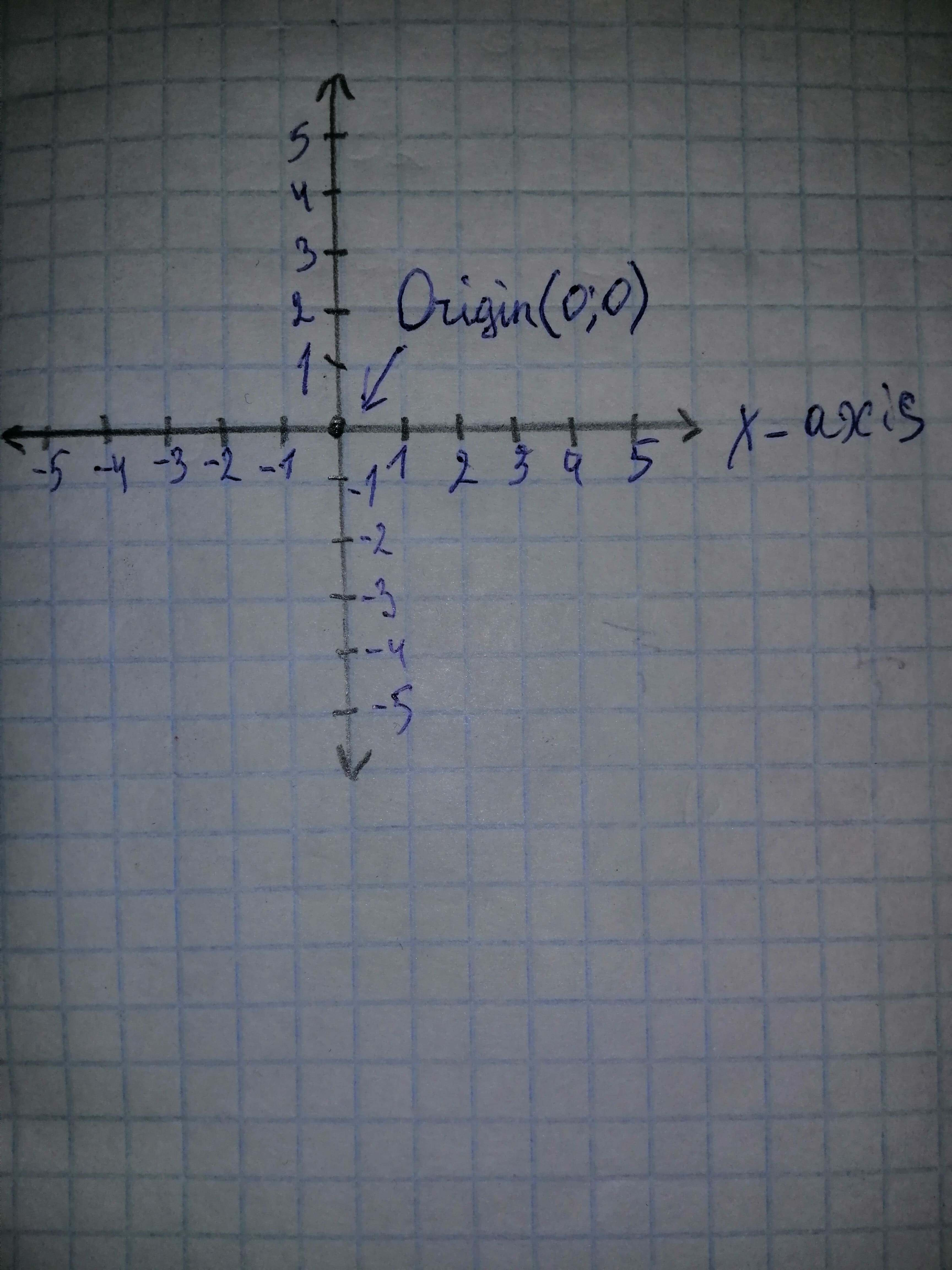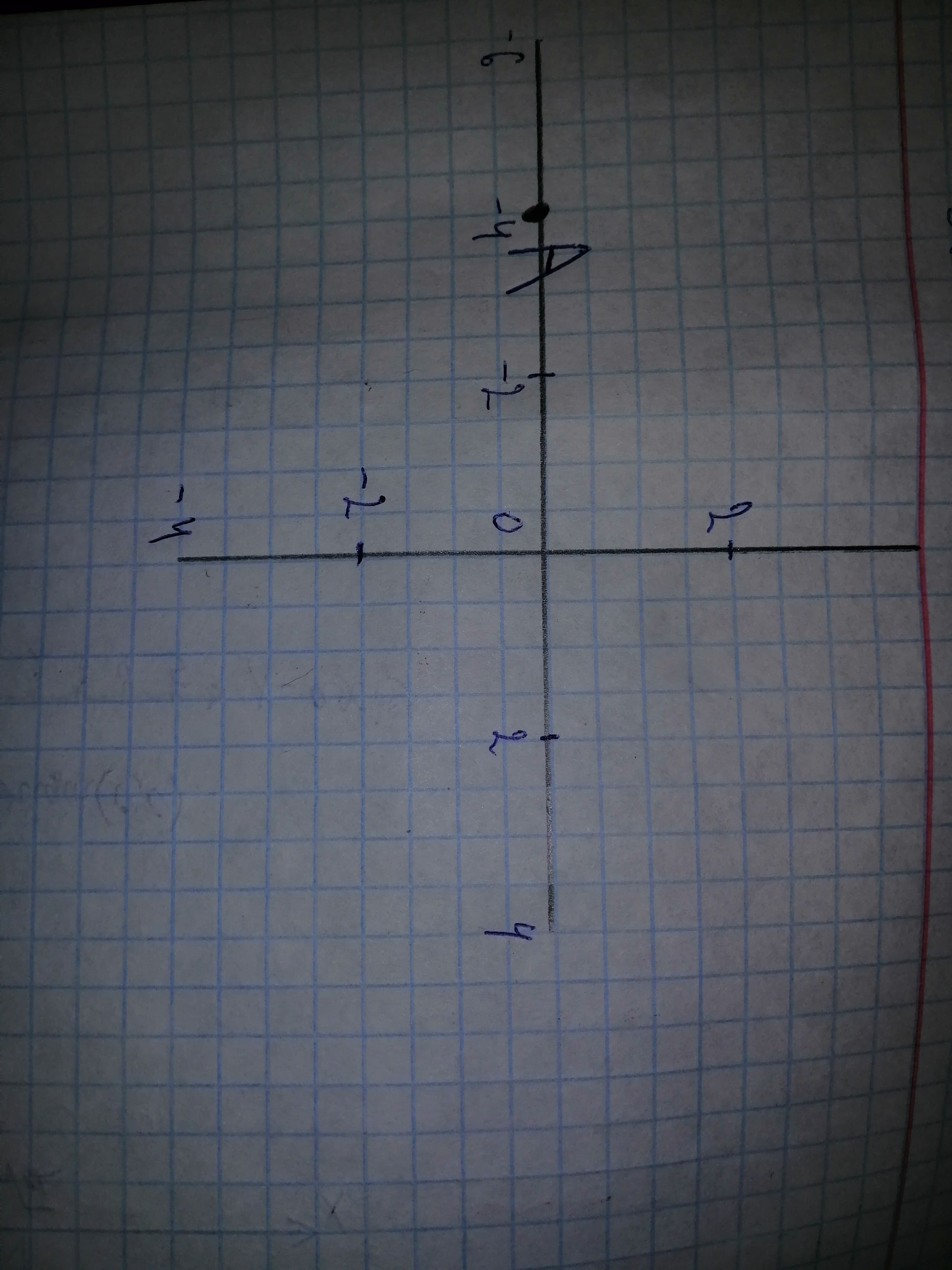# Plot the given point (-4, 0) in a rectangular coordinate system.Ernstfalld 2021-01-28 Answered
Plot the given point $\left(-4,0\right)$ in a rectangular coordinate system.
You can still ask an expert for help

• Questions are typically answered in as fast as 30 minutes

Solve your problem for the price of one coffee

• Math expert for every subject
• Pay only if we can solve ithajavaF

Step 1
The rectangular coordinate system consists of two real number lines that intersect at a right angle.
The horizontal number line is called the x-axis, and the vertical number line is called the y-axis.
These two number lines define a flat surface called a plane, and each point on this plane is associated with an ordered pair of real numbers (x, y). The first number is called the x-coordinate, and the second number is called the y-coordinate.
The intersection of the two axes is known as the origin, which corresponds to the point (0, 0)Step 2
An ordered pair (x, y) represents the position of a point relative to the origin.
The x-coordinate represents a position to the right of the origin if it is positive and to the left of the origin if it is negative. The y-coordinate represents a position above the origin if it is positive and below the origin if it is negative.
Using this system, every position (point) in the plane is uniquely identified. For example, the we have pair (-4, 0) denotes the position relative to the origin as shown:Thus, point A is shown in the above graph.